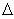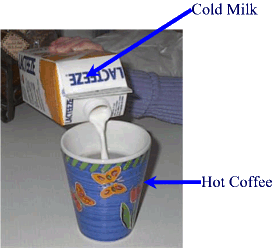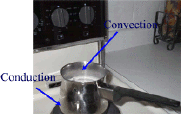#### Kinetic Molecular Theory of Heat

The Particle Theory of matter explains that all matter is made up of particles and that all particles are always in constant motions.

 Solids - Vibration Energy Liquids - Vibration & Translational Energies Gases - Vibration, Rotational, Translational Energies

#### Temperature Scales.

##### The Celsius (or centigrade) scale:

It can be mathematically proven that temperature is the average kinetic energy of the particles of a substance.  Temperature scales have been devised around common, arbitrary reference points as to the state of common substances.  Water is one of these common substances.  For example, the freezing point and the boiling point of water respectively were chosen to indicate zero degrees (00C) and one hundred degrees Celsius (1000C).  Because this scale goes from zero to one hundred degrees it is also known as the centigrade scale - from the latin word "cento" which means one hundred .  Normal average human body temperature was then standardized at 370C, and room temperature is taken to be 200C.

##### The Kelvin (or absolute) scale:

From a physics point of view, it makes more sense to relate the temperature of a substance to the motion of the molecules within that substance and thus their kinetic energy.An "ideal" absolute temperature of ZERO cannot be reproduced in a lab.  This point, known as the absolute zero or ZERO KELVIN (after Lord Kelvin) is calculated by extrapolating and interpreting the cooling curves of common substances.

A cooling curve is a temperature-time graph taken of a substance as it is cooling and undergoing changes of state from a vapour to a solid.

Consider the cooling curve of water belowNote that Water freezes at 00C.

To bring the motion of the water (ice) molecules to a complete stop the temperature needs to be dropped down to -273 0C. This point is known as absolute zero or 0 K (Zero Kelvin). On the Kelvin scale water boils at 373 K an freezes at 273 K.  Room temperature (200C.) is 293 K and STP (Standard Temperature) will have a reading of 298 K. To convert from Celsius to Kelvin simply add 273 to the Celsius temperature.Note that a change in Celsius temperature is equivalent to a change in Kelvin degrees.  For example from 200C. to 600C there is a temperature  change of 400C..  This is the same amount of change in K.

#### Heating Curves

The reverse of a cooling curve is called a heating curve.  Heating curves and cooling curves can explain what happens to a substance in terms of temperature changes, and heat content of the particles of the substance when they undergo changes of state from solid, to liquid, to gas (vapour state) and vice versa. An exothermic process is a process that produces heat while an endothermic process will require the addition of heat to proceed.

##### Exercise:

1. Change the following in Kelvin (K):

a) 2 0C = _________________ K

b) -132 0C = ______________  K

2. Change the following in  0C

a) 100 K = __________________0C

b) 30 K = _________________ 0C

c) 500 K =  _________________ 0CSolutions

#### Specific Heat Capacity

Some substance have a heat content which is naturally higher than others.  For example you can walk on grass on a hot day but you probably will not be able to walk on a sandy beach with the same amount of comfort on the same day.  Sand has a higher specific heat capacity than grass.

The heat content (Q) of an object can be calculated in terms of :

• its mass (m)
• its temperature change (DT)
• and its specific heat capacity (c)

We ca summarize the above in the following  mathematical expression:

Q = m x c xT

The specific heat capacity of a substance is defined as the amount of heat energy that must be added or removed from a substance to change the temperature of 1 kg of the substance by 1 K (Kelvin degree).

##### Exercise:

A. A 700 g  aluminum kettle (caluminum = 920 J/Kg/k)  is left to cool off for 10 minutes after boiling water.  Its final temperature reaches 350C.  Assuming that its initial temperature was that of boiling water (100 0C), how much heat energy did the kettle lose in 10 min?

B. If it took 4 minutes for the kettle to boil 500 mL of water (cwater = 4200 J/Kg/k) from 200C to 1000C  what is the power of the kettle?  [Density of water = 1g/mL]

#### Principle of Heat Exchange

This principle is basically a restatement of the Law of Conservation of Energy.  It says that during a process where an object or substance gains heat at the expense of another substance, the amount of heat gained by the colder substance equals the amount of heat lost by the warmer substance.  This is an idealized situation because in the real world it can only be applied if we consider a 100% closed system where no energy (heat) escapes to the outside or the surroundings.  In symbols:

Qlost = Qgained##### Exercise:

To cool down a 200 mL cup of coffee at 650C, you add 15.0 mL of milk at 10.0 0C.  What is the final temperature of  the coffee.  The specific heat capacity of milk is 3700 J/K.Kg, the specific heat capacity of  coffee is 4200 J/K.Kg,  [For coffee and milk assume that the densities are the same as water. i.e.: D =1.0 g/mL]

Transmission of Heat

Heat is transmitted primarily in three different ways: conduction, convection, and radiation.   Conduction is a slow process where the molecules of an object transfer heat by "touching" those of a colder object.  This method requires the presence of a medium and it is the typical transfer method of heat between solids and liquids or both.  Convection occurs when the "hot" molecules of an object transfer the energy to the air molecules surrounding it and in turn these will carry the heat to the cooler spaces beyond.  Convection requires the presence of a medium as well and cannot occur in empty space or vacuums.   Convection occurs usually between (fluids) liquids and gases or liquids and other liquids  Radiation can take place where a hot object send heat in the form electromagnetic waves through empty space or through a vacuum.  This is the case of the heating effect between the Sun and the Earth.

• Conduction: occurs between the hot stove element and the cooler bottom of the pot
• Convection: occurs between the hot milk (water vapour) and the cooler air molecules surrounding the top of the milk surface#### Latent Heats

The word latent refers to the "hidden" or intrinsic heat found within the molecules of solids or liquids as the energy required to keep these molecules bonded in the solid or liquid states.  When a solid melts or a liquid vapourizes this energy is liberated in the form of heat.   The higher the mass of a substance the higher the stored heat or latent heat.  Different substances have different amounts of energy.

The latent heat of fusion (Q-- for melting)  can be calculated using the following equation:

Q= lx m

In the same way the latent heat of vapourization (Q-- for a liquid turning into a gas or vapour)  can be calculated using the following equation:

Q= lx m

The constants land lare known as the latent heats of fusion and vapourization respectively.

##### Exercise:

The heat of vapourization of water is 2400 KJ/Kg.  On a hot day (400C) a 70 kg man sweats so that 160 W of power are dissipated from his skin.   How much sweat does he lose per hour through evapouration from his skin?

##### Exercise:

How much ice (-50C) is needed to bring down the temperature of a tub of water (50 L) from 700C to 200C.  Assume the ice melts in the process.  [lf ice = 335 KJ/Kg; lv water = 2260 KJ/Kg]